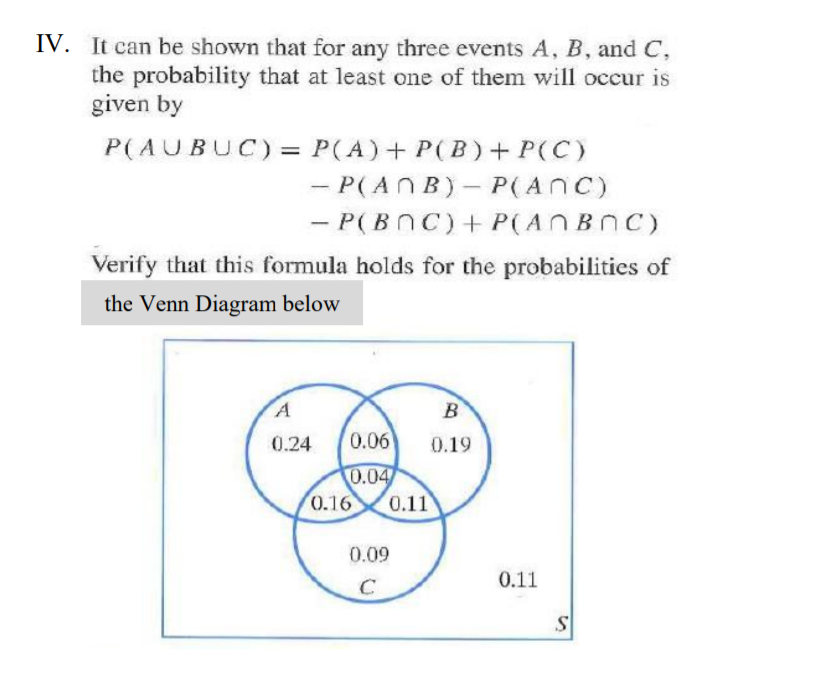# Question IV. It can be shown that for any three events A, B, and C. the probability that at least one of them will occur is given by P(AUBUC)-P(A) PB)P(C) Verify that this formula holds for the probabilities of the Venn Diagram below 0.24 0.06 0.19 0.0 0.160.11 0.09 0.118HVZZV The Asker · Other MathematicsTranscribed Image Text: IV. It can be shown that for any three events A, B, and C. the probability that at least one of them will occur is given by P(AUBUC)-P(A) PB)P(C) Verify that this formula holds for the probabilities of the Venn Diagram below 0.24 0.06 0.19 0.0 0.160.11 0.09 0.11
More
Transcribed Image Text: IV. It can be shown that for any three events A, B, and C. the probability that at least one of them will occur is given by P(AUBUC)-P(A) PB)P(C) Verify that this formula holds for the probabilities of the Venn Diagram below 0.24 0.06 0.19 0.0 0.160.11 0.09 0.11